In the June, 2020, issue I featured three books on this very topical subject, written (or co-authored) by Canadian researchers. Three of the five books presented in this column go a bit further afield, but Ping Yan, co-author of “Quantitative Methods for Investigating Infectious Disease Outbreaks” is a Research Manager at the Public Health Agency of Canada, and Robert Smith?, author of “Modelling Disease Ecology with Mathematics”, is a Professor of Biomathematics at the University of Ottawa. In the interest of a timely publication of this column, only the publishers’ descriptions, rather than actual reviews, are provided below.

Karl Dilcher, Dalhousie University (notes-reviews@cms.math.ca)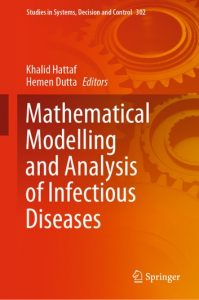Mathematical Modelling and Analysis of Infectious Diseases
Edited by K. Hattaf and H. Dutta

Studies in Systems, Decision and Control 302, Springer, 2020
ISBN 978-3-03049895-5

Publisher’s Description:

This book discusses significant research and study topics related to mathematical modelling and analysis of infectious diseases. It includes several models and modelling approaches with different aims, such as identifying and analysing causes of occurrence and re-occurrence, causes of spreading, treatments and control strategies. A valuable resource for researchers, students, educators, scientists, professionals and practitioners interested in gaining insights into various aspects of infectious diseases using mathematical modelling and mathematical analysis, the book will also appeal to general readers wanting to understand the dynamics of various diseases and related issues.

Key Features

• Mathematical models that describe population prevalence or incidence of infectious diseases
• Mathematical tools and techniques to analyse data on the incidence of infectious diseases
• Early detection and risk estimate models of infectious diseases
• Mathematical models that describe the transmission of infectious diseases and analyse data
• Dynamical analysis and control strategies for infectious diseases
• Studies comparing the utility of particular models in describing infected diseases-related issues such as social, health and economic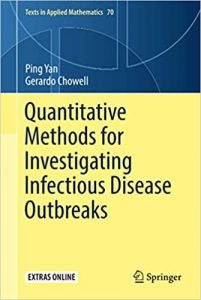Quantitative Methods for Investigating Infectious Disease Outbreaks
By P. Yang and G. Chowell
Texts in Applied Mathematics 70, Springer, 2019
ISBN 978-3-030-21922-2

Publisher’s Description:

This book provides a systematic treatment of the mathematical underpinnings of work in the theory of outbreak dynamics and their control, covering balanced perspectives between theory and practice including new material on contemporary topics in the field of infectious disease modelling. Specifically, it presents a unified mathematical framework linked to the distribution theory of non-negative random variables; the many examples used in the text, are introduced and discussed in light of theoretical perspectives.

The book is organized into 9 chapters: The first motivates the presentation of the material on subsequent chapters; Chapter 2-3 provides a review of basic concepts of probability and statistical models for the distributions of continuous lifetime data and the distributions of random counts and counting processes, which are linked to phenomenological models. Chapters 4 focuses on dynamic behaviors of a disease outbreak during the initial phase while Chapters 5-6 broadly cover compartment models to investigate the consequences of epidemics as the outbreak moves beyond the initial phase. Chapter 7 provides a transition between mostly theoretical topics in earlier chapters and Chapters 8 and 9 where the focus is on the data generating processes and statistical issues of fitting models to data as well as specific mathematical epidemic modeling applications, respectively.

This book is aimed at a wide audience ranging from graduate students to established scientists from quantitatively oriented fields of epidemiology, mathematics and statistics. The numerous examples and illustrations make understanding of the mathematics of disease transmission and control accessible. Furthermore, the examples and exercises, make the book suitable for motivated students in applied mathematics, either through a lecture course, or through self-study.  This text could be used in graduate schools or special summer schools covering research problems in mathematical biology.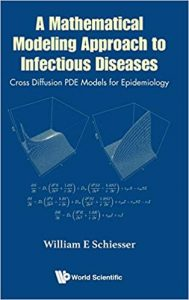A Mathematical Modeling Approach to Infectious Diseases
Cross Diffusion PDE Models for Epidemiology
By W. E. Schiesser
World Scientific, 2018
ISBN 978-981-3238-78-7

Publisher’s Description:

The intent of this book is to provide a methodology for the analysis of infectious diseases by computer-based mathematical models. The approach is based on ordinary differential equations (ODEs) that provide time variation of the model dependent variables and partial differential equations (PDEs) that provide time and spatial (spatiotemporal) variations of the model dependent variables.

The starting point is a basic ODE SIR (Susceptible Infected Recovered) model that defines the S,I,R populations as a function of time. The ODE SIR model is then extended to PDEs that demonstrate the spatiotemporal evolution of the S,I,R populations. A unique feature of the PDE model is the use of cross diffusion between populations, a nonlinear effect that is readily accommodated numerically. A second feature is the use of radial coordinates to represent the geographical distribution of the model populations.

The numerical methods for the computer implementation of ODE/PDE models for infectious diseases are illustrated with documented R routines for particular applications, including models for malaria and the Zika virus. The R routines are available from a download so that the reader can reproduce the reported solutions, then extend the applications through computer experimentation, including the addition of postulated effects and associated equations, and the implementation of alternative models of interest.

The ODE/PDE methodology is open ended and facilitates the development of computer-based models which hopefully can elucidate the causes/conditions of infectious disease evolution and suggest methods of control.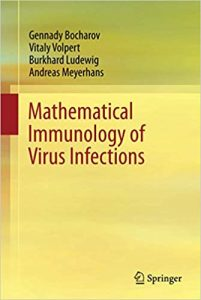Mathematical Immunology of Virus Infections
By G. Bocharov, V. Volpert, B. Ludewig, and A. Meyerhans
Springer, 2018
ISBN: 978-3-319-72316-7

Publisher’s Description:

This monograph concisely but thoroughly introduces the reader to the field of mathematical immunology. The book covers first basic principles of formulating a mathematical model, and an outline on data-driven parameter estimation and model selection. The authors then introduce the modeling of experimental and human infections and provide the reader with helpful exercises. The target audience primarily comprises researchers and graduate students in the field of mathematical biology who wish to be concisely introduced into mathematical immunology.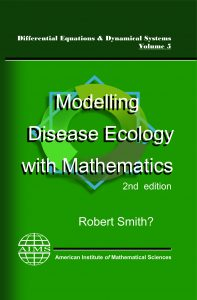Modelling Disease Ecology with Mathematics, 2nd Ed.
By Robert Smith?
AIMS Series on Differential Equations & Dynamical Systems, 5
American Institute of Mathematical Sciences, 2017
ISBN: 978-1-60133-020-8

Publisher’s Description:

“Modelling Disease Ecology with Mathematics” is a self-contained introduction to the basics of mathematics for students and researchers in the areas of biology, epidemiology, medicine and public health. Diseases covered include malaria, yellow fever, measles, influenza, Guinea-worm disease and AIDS.

a) Mathematical models representing current diseases are formulated and analysed in an easy-to-follow manner, often humorously.

b) MATLAB exercises provide the reader with the ability to develop control strategies, test hypothetical interventions and explore disease-management options.

c) Case studies provide worked examples of applying theoretical tools to real-life problems (and also prepare the world for a zombie apocalypse).

This monograph is especially suited to those without a background in mathematics, who are interested in learning about the way that mathematics can organise, analyse and enlighten when tackling biological problems in disease control and management.